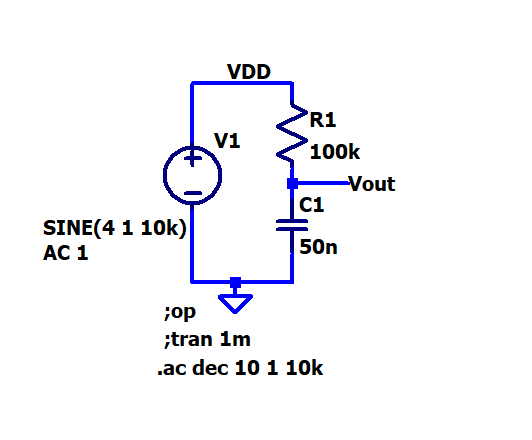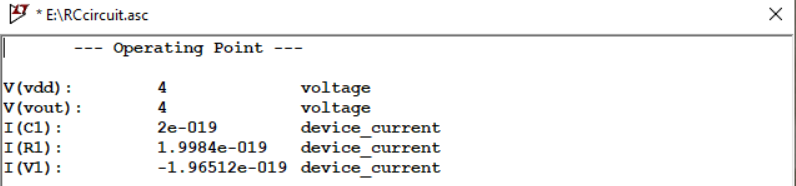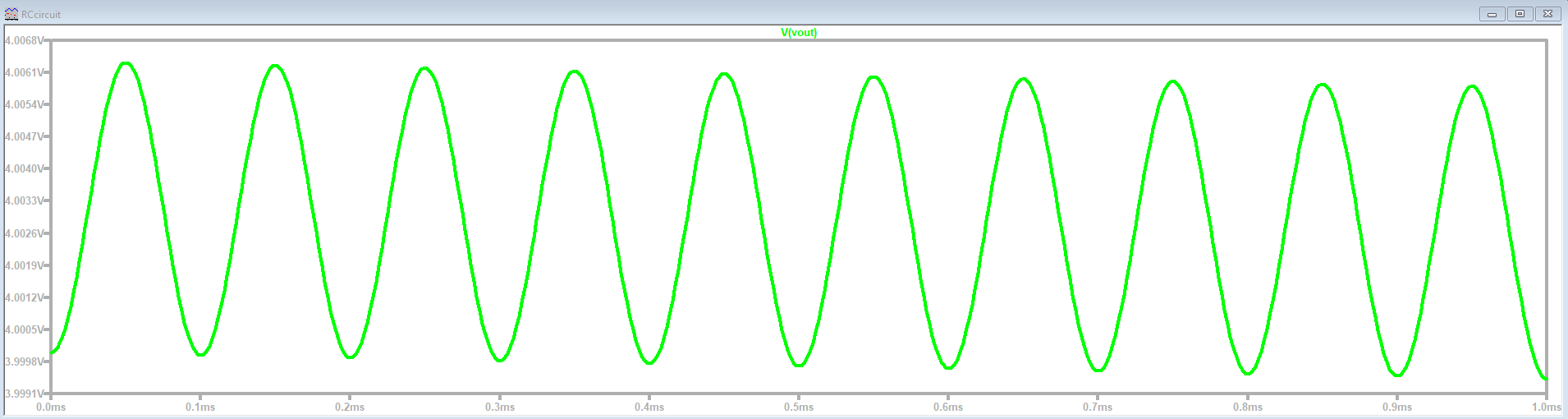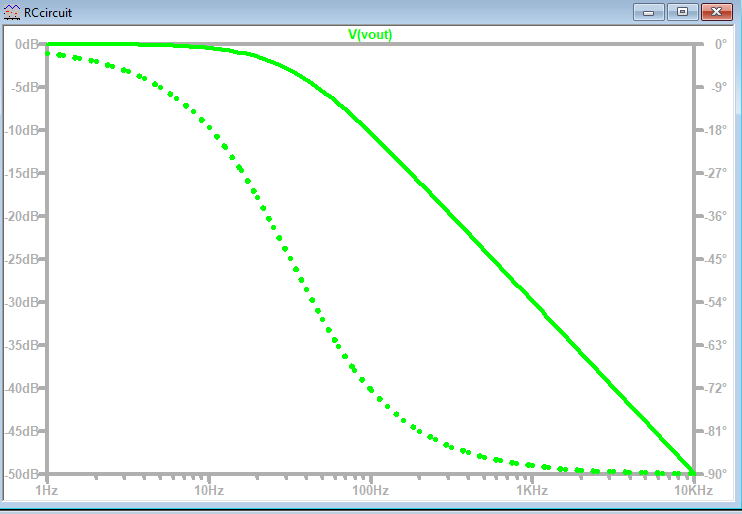# Laboratory Goals

The objectives of this laboratory session are as follows:

• Introduction to LTspice
• Designing a Low pass with 3dB and simulating it.
• Introduction to creation of a web report.
• Preparation for the simulation of a data convertor.

# Introduction

• LTspice: is a circuit simulator used to simulate circuits before the real implementation.

• Using a web page to publish a report is possible by editing a raw html code. It is also possible to insert LTspice schematics to the web page since a code was written by the instructor of this subject which is capable to read a LTspice schematic file and present the schematic in the web page.

# LTspice Low Pass Filter

 The following LTspice schematic of a low pass filter was designed and simulated with a voltage source (sine,AC), R = 100k and C1 = 50 n, with three different simulation commands (.Op, .tran, .AC) :

# LTspice Low Pass Filter

 The following figure shows the designed and simulated low pass filter circuit:figure 1

# Low Pass Filter Operating Point

 The following figure shows the operating point of the simulated low pass filter.figure 2

# Low Pass Filter Simulation

 The following figure shows the transient output of the simulated low pass filter.figure 3

# Low Pass Filter Simulation

 The following figure shows the simulated output of the low pass filter.figure 4
From the simulation result, it is clear that the low pass filter characterstics matches the simulation output.

# Challenges and Difficulties

While implementing this laboratory session, some challenges and difficulties were faced. These challenges and diffeculties are listed below:

• Downloading and unzipping the (web_Template.zip) folder needed some time.
• Getting to know how to edit the raw web page code and being able to pulish a report was a big challenge.

# Lessons Learned and Time Spent

The following lessons were learned out of this lab:

• Simulation using LTSPICE.
• The following three commands in LTspice:
• .op Simulation command for operation point.
• .tran simulate over time period T=1/f to see a couple of periods.
• AC simulates a frequency sweep.
• Many commands to edit the structure of the web page were learned include:
• Specifying the sections of the document
• The tab name in the browser.
• Adding comments.
• A section for a new slide.
• A header in a slide section.
• Writing a text inside a slide section.
• Inserting pictures from a folder.
• Inserting a LTspice schematic.

The time spent working on this lab was around 8 hours distributed between the lab sessions and the working on the report.

# Conclusion

From this laboratory session, we conclude the following:

• Notepad++ can be used to edit the raw html code for the webpage
• A low pass filter was designed and simulated in LTspice using three different simulation commands.
• There was a need to modify the configurations for the privacy of the web page.

# Clarification

The work on this lab session including the simulation implementation and work on the report was done in group. The student with the identification number ***726 implemented the simulation, while the student with the identification number ***910 created this web report.